# 「R」数据可视化5：PCA和PCoA图

2020-01-13 1167动手实验室

## 什么是PCA和PCoA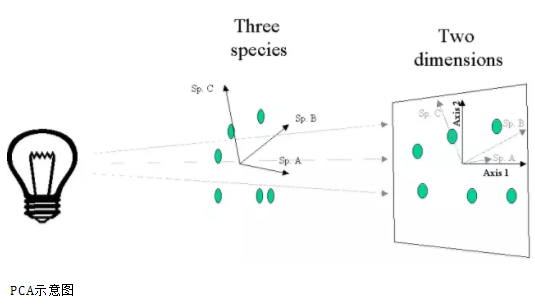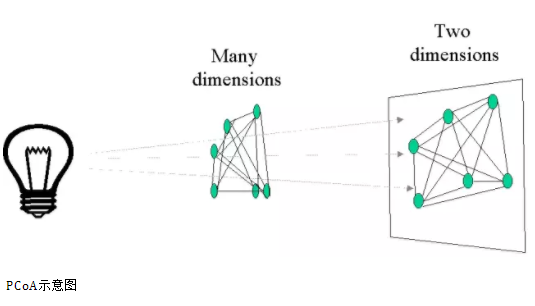## 如何进行PCA和PCoA分析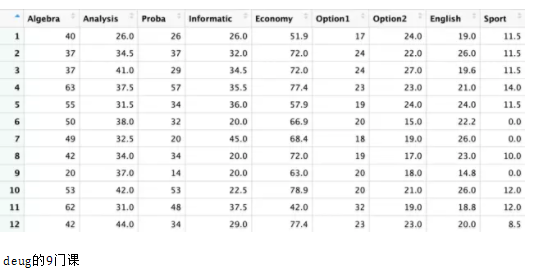### PCA分析及作图

library(ggplot2)
library(RColorBrewer)
data(deug)

# PCA分析

pca<- dudi.pca(deug\$tab, scal = FALSE, center = deug\$cent, scan = FALSE)

# 坐标轴解释量（前两轴）

pca_eig <- (pca\$eig)[1:2] / sum(pca\$eig)

# 提取样本点坐标（前两轴）

sample_site <- data.frame({pca\$li})[1:2]
sample_site\$names <- rownames(sample_site)
names(sample_site)[1:2] <- c('PCA1', 'PCA2')

# 以最终成绩作为分组sample_site\$level<-factor(deug\$result,levels=c('A+','A','B','B-','C-','D'))

library(ggplot2)

pca_plot <- ggplot(sample_site, aes(PCA1, PCA2,color=level)) +theme_classic()+#去掉背景框
geom_vline(xintercept = 0, color = 'gray', size = 0.4) +
geom_hline(yintercept = 0, color = 'gray', size = 0.4) +
geom_point(size = 1.5)+ #可在这里修改点的透明度、大小
scale_color_manual(values = brewer.pal(6,"Set2")) + #可在这里修改点的颜色
theme(panel.grid = element_line(color = 'gray', linetype = 2, size = 0.1),
panel.background = element_rect(color = 'black', fill = 'transparent'),
legend.title=element_blank()
)+
labs(x = paste('PCA1: ', round(100 * pca_eig, 2), '%'), y = paste('PCA2: ', round(100 * pca_eig, 2), '%'))
pca_plot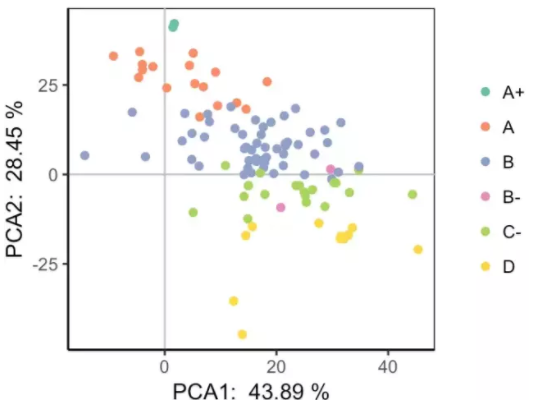### PCoA分析及作图

library(ggplot2)
library(RColorBrewer)
library(vegan)#用于计算距离
data(deug)
tab<-deug\$tab
tab.dist<-vegdist(tab,method='euclidean')#基于euclidean距离
pcoa<- dudi.pco(tab.dist, scan = FALSE,nf=3)

# 坐标轴解释量（前两轴）

pcoa_eig <- (pcoa\$eig)[1:2] / sum(pcoa\$eig)

# 提取样本点坐标（前两轴）

sample_site <- data.frame({pcoa\$li})[1:2]
sample_site\$names <- rownames(sample_site)
names(sample_site)[1:2] <- c('PCoA1', 'PCoA2')

# 以最终成绩作为分组

sample_site\$level<-factor(deug\$result,levels=c('A+','A','B','B-','C-','D'))

library(ggplot2)

pcoa_plot <- ggplot(sample_site, aes(PCoA1, PCoA2,color=level)) +
theme_classic()+#去掉背景框
geom_vline(xintercept = 0, color = 'gray', size = 0.4) +
geom_hline(yintercept = 0, color = 'gray', size = 0.4) +
geom_point(size = 1.5)+ #可在这里修改点的透明度、大小
scale_color_manual(values = brewer.pal(6,"Set2")) + #可在这里修改点的颜色
theme(panel.grid = element_line(color = 'gray', linetype = 2, size = 0.1),
panel.background = element_rect(color = 'black', fill = 'transparent'),
legend.title=element_blank()
)+
labs(x = paste('PCoA1: ', round(100 * pcoa_eig, 2), '%'), y = paste('PCoA2: ', round(100 * pcoa_eig, 2), '%'))
pcoa_plot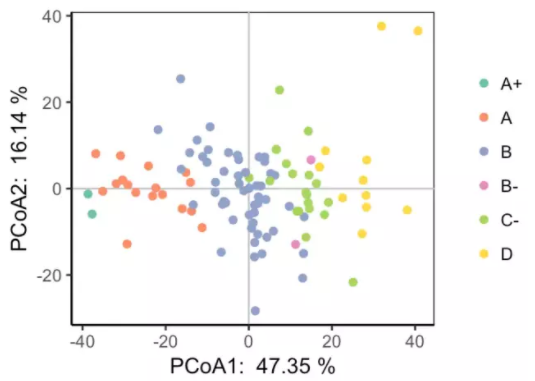「R」数据可视化4: 金字塔图和偏差图

「R」数据可视化3 : 热图

「R」数据可视化2 : 箱形图

「R」数据可视化1: 火山图

▍本文版权（包括图片及文字）属于“优雅R”（微信公众号：elegant-r），禁止二次转载，如需转载请联系w_shixiang@163.com部分数据和图片来源于网络，如有版权问题请联系删除。